# NCERT Solutions class-11 Maths Exercise 2.3## myCBSEguide App

CBSE, NCERT, JEE Main, NEET-UG, NDA, Exam Papers, Question Bank, NCERT Solutions, Exemplars, Revision Notes, Free Videos, MCQ Tests & more.

Exercise 2.3

1. Which of the following are functions? Give reasons. If it is a function determine its domain and range.

(i) {(2, 1), (5, 1), (8, 1), (11, 1), (14, 1), (17, 1)}

(ii) {(2, 1), (4, 2), (6, 3), (8, 4), (10, 5), (12, 6), (14, 7)}

(iii) {(1, 3), (1, 5), (2, 5)}

Ans. (i) Given: Relation is {(2, 1), (5, 1), (8, 1), (11, 1), (14, 1), (17, 1)}

All values of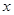are distinct. Each value ofhas a unique value of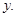Therefore, the relation is a function.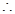Domain of function = {2, 5, 8, 11, 14, 17}

Range of function = {1}

(ii) Given: Relation is {(2, 1), (4, 2), (6, 3), (8, 4), (10, 5), (12, 6), (14, 7)}

All values ofare distinct. Each value ofhas a unique value ofTherefore, the relation is a function.Domain of function = {2, 4, 6, 8, 10, 12, 14}

Range of function = {1, 2, 3, 4, 5, 6, 7}

(iii) Given: Relation is {(1, 3), (1, 5), (2, 5)}

This relation is not a function because there is an element 1 which is associated to two elements 3 and 5.

2. Find the domain and range of the following real functions:

(i)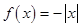(ii)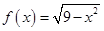Ans. (i) Given:. The function is defined for all real values of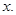Domain of the function = R

Now, when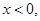then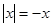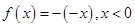When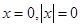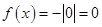When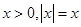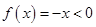Therefore,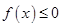for all real values ofRange of function =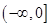(ii) Given:.

The function is not defined when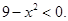Domain of function =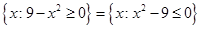=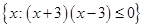=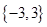Range of function =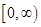3. A function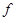is defined by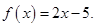Write down the values of:

(i)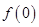(ii)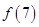(iii)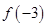Ans. Given: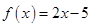(i) Putting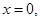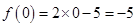(ii) Putting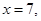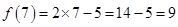(iii) Putting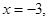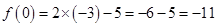4. The function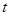which maps temperature in degree Celsius into temperature in degree Fahrenheit is defined by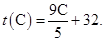Find:

(i)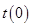(ii)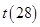(iii)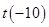(iv) The value of C when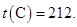Ans. Given:(i) Putting C = 0,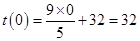(ii) Putting C = 28,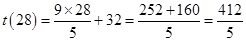(iii) Putting C = –10,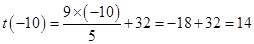(iv) Putting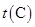= 212,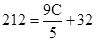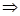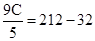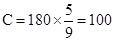5. Find the range of each of the following functions:

(i)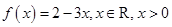(ii)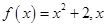is a real number.

(iii)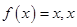is a real number.

Ans. (i) Given: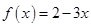,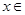R and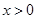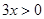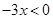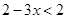Range of function

=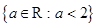=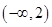(ii) Given: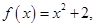R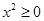forR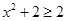Range of function

=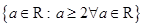=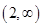(iii) Given: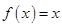,RRange of function = R### 3 thoughts on “NCERT Solutions class-11 Maths Exercise 2.3”

1. I cant understand 2nd question 2nd part

2. you haven’t even explained the questions in which you take out the range

3. 2nd question kuch vi smj ni aaya..